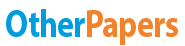# Ampere's Law

Essay by   •  November 14, 2011  •  Essay  •  847 Words (4 Pages)  •  1,413 Views

## Essay Preview: Ampere's Law

Report this essay
Page 1 of 4

OBJECTIVE

The goal of this experiment is to show the validity of Ampere's Law while learning about the application of mageto-resistive sensors. This experiment measures the magnetic field strength as a function of the distance measured perpendicularly from a long current carrying wire.

INTRODUCTION

Theory:

Static magnetic fields contain currents flowing through wires or space, and in geometrically symmetric situations. Ampere's Law enables the calculation of the magnetic field in terms of current. Ampere's Law is a mathematical formulation that relates the magnetic field strength with any currents associated with that magnetic field. It is the closed integration of the magnitude of the magnetic field that equals the product between the permeability of free space and the current expressed

∮▒〖Bdl =B∮▒dl= μ_0 i 〗 --- (1)

Integration along the length of the circle yields the infinitesimal differences of the following equation, where B and l are vector quantities:

∑▒〖B ∙ ∆l〗 = μ_0 i --- (2)

In general, B and ∆l have, a product that only the component of B is multiplied times the increment length. In vector multiplication, B ∙ ∆l is the "dot product" or the scalar multiplication given by

B ∙ ∆l = B∆l cosθ =Bcosθ∆l --- (3),

where θ is the angle between B and ∆l.

The current on the wire generates a magnetic field that follows the right-hand rule. If the magnetic field moves clockwise, the current flows into the board. The converse is also true, where if the current were moving into the paper, the magnetic field would be clockwise.

If the circular path was divide into a large number of increments, ∆l as shown in equation (3), B has the same magnitude at every increment and is in the same direction as ∆l. Here, the angler between B and ∆l is zero. Using θ = 0o, and cosθ = 1, the product B*∆ is B∆l. thus the summation expression is now

∑▒〖B ∙ ∆l〗 = ∑▒〖B ∙ ∆l cos0〗 = ∑▒B∆l =μ_0 i --- (4)

Where ∑▒∆l =2πr, and B2πr = μ_0 i

Therefore,

B = (μ_0 i)/2πr --- (5)

After rearranging the equation the function:

B/i = μ_0/2π 1/r --- (6)

gives B⁄i a linear function of 1⁄r to give a straight line when graphed.

The very long straight length of wire carrying a current verifies Ampere's law using this relationship. The magnetic field decreases inversely with distance and measured outward from the wire.

EXPERIMENT SET UP

Apparatus:

The list of apparatus consists of the following items:

Giant Magneto Resistive (GMR) sensor

The distance in the apparatus is determined with a digital scale to a precision of 0.01 mm and the radius is the distance between the centre of the wire and the point that the sensor is located beneath the surface of its mounting package. The Giant Magneto Resistive (GMR) sensor measured the magnetic field whose change in volts is directly proportional to the change in magnetic field strength.

Procedure:

The digital ammeter determines the measure of the current present in the wire. Data was obtained at approximately 0.1 mm intervals in the range 0.2 mm to 1.00, 0.5 intervals in the range 1 mm to 5 mm, 1.0 mm intervals in the range 5 mm to 10 mm, and 5.0 mm intervals in the range 10 mm to 50 mm.

After collecting data, a graph of B⁄i versus 1⁄r was plotted to allow acquisition of the linear regression equation. The μ_0 value was measured. The slope (m) of this line gives the value of μ_0/2π .

The measured value and theoretical value of μ_0 were compared to verify Ampere's Law.

RESULTS

r' (m) Sensor depth

s (m) Position of GMR sensor

p Voltage without current

V0 Voltage current present

V Current

I (A) 1/r

= 1/(r'+s+p)

(1/m) ΔV/I

=(V-V_0)/i K

B/I

=k∆V/i

1.18E-03 5.00E-04 0.21 1.337 11.77 7.43 4.72E+00 1.404172 7.31E-05 1.03E-04

...

...